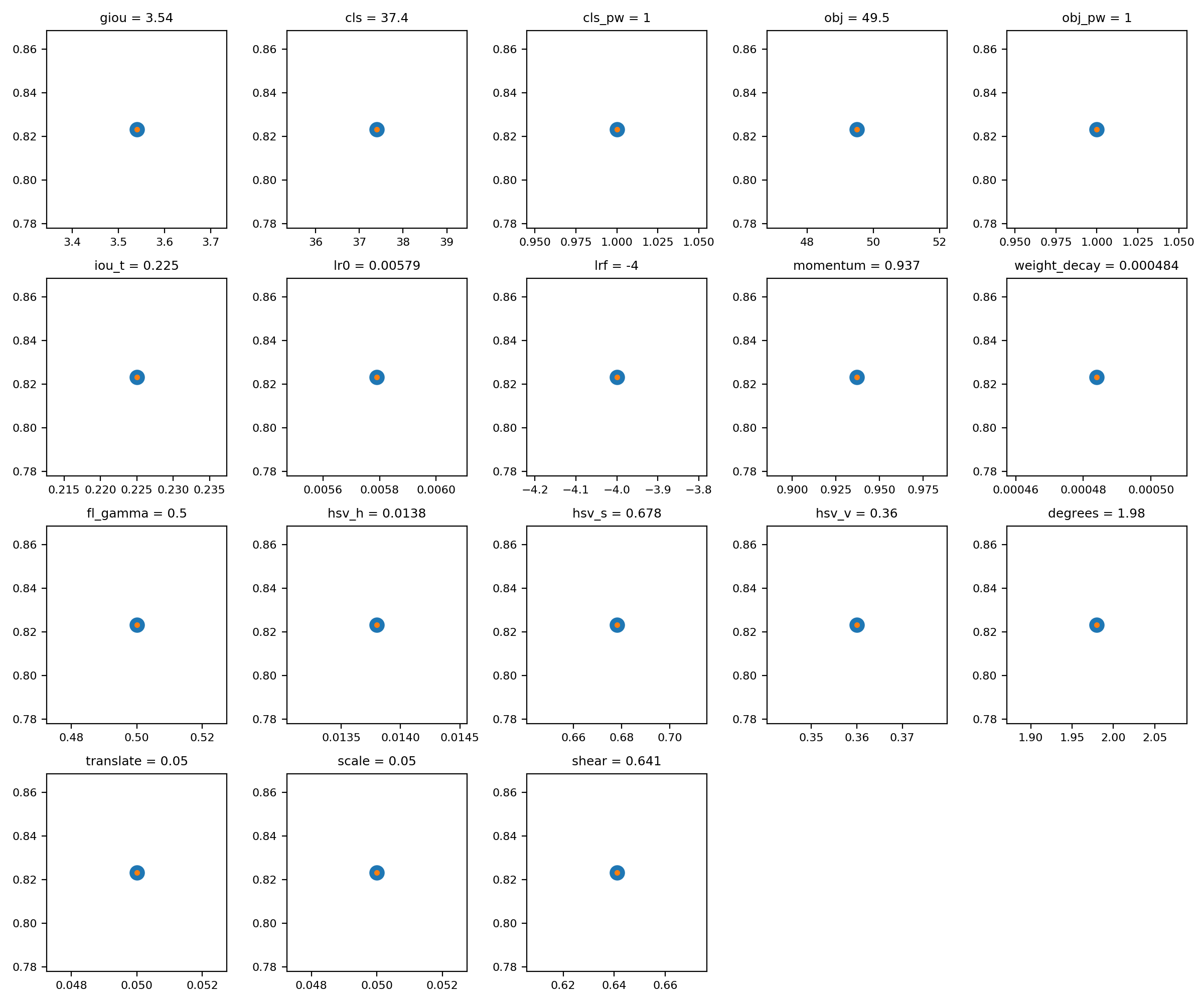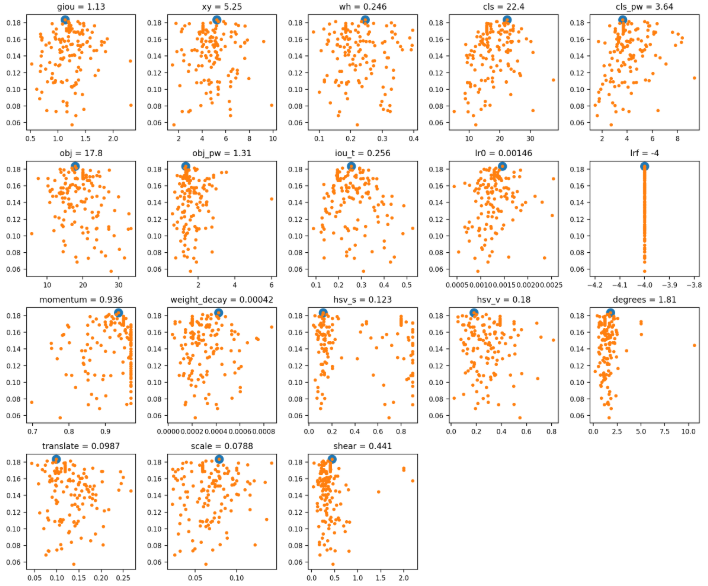# 【从零开始学习YOLOv3】4. YOLOv3中的参数搜索¶

## 1. 超参数¶

YOLOv3中的 超参数在train.py中提供，其中包含了一些数据增强参数设置，具体内容如下：

hyp = {'giou': 3.54,  # giou loss gain
'cls': 37.4,  # cls loss gain
'cls_pw': 1.0,  # cls BCELoss positive_weight
'obj': 49.5,  # obj loss gain (*=img_size/320 if img_size != 320)
'obj_pw': 1.0,  # obj BCELoss positive_weight
'iou_t': 0.225,  # iou training threshold
'lr0': 0.00579,  # initial learning rate (SGD=1E-3, Adam=9E-5)
'lrf': -4.,  # final LambdaLR learning rate = lr0 * (10 ** lrf)
'momentum': 0.937,  # SGD momentum
'weight_decay': 0.000484,  # optimizer weight decay
'fl_gamma': 0.5,  # focal loss gamma
'hsv_h': 0.0138,  # image HSV-Hue augmentation (fraction)
'hsv_s': 0.678,  # image HSV-Saturation augmentation (fraction)
'hsv_v': 0.36,  # image HSV-Value augmentation (fraction)
'degrees': 1.98,  # image rotation (+/- deg)
'translate': 0.05,  # image translation (+/- fraction)
'scale': 0.05,  # image scale (+/- gain)
'shear': 0.641}  # image shear (+/- deg)


## 2. 使用方法¶

python train.py --data data/voc.data
--cfg cfg/yolov3-tiny.cfg
--img-size 416
--epochs 273
--evolve


for _ in range(1):  # generations to evolve## 3. 原理¶

s = 0.2 # sigma


1. 如何评判其中一代的好坏？
2. 下一代如何根据上一代进行进化？

def fitness(x):
w = [0.0, 0.0, 0.8, 0.2]
# weights for [P, R, mAP, F1]@0.5
return (x[:, :4] * w).sum(1)


YOLOv3进化部分是通过以上的适应度函数判断的，适应度越高，代表这一代的性能越好。而在适应度中，是通过Precision,Recall ,mAP,F1这四个指标作为适应度的评价标准。

 if parent == 'single' or len(x) == 1:
x = x[fitness(x).argmax()]


elif parent == 'weighted':  # weighted combination
n = min(10, len(x))  # number to merge
x = x[np.argsort(-fitness(x))][:n]  # top n mutations
w = fitness(x) - fitness(x).min()  # weights
x = (x * w.reshape(n, 1)).sum(0) / w.sum()  # new parent


# Mutate
method = 2
s = 0.2  # 20% sigma
np.random.seed(int(time.time()))
g = np.array([1, 1, 1, 1, 1, 1, 1, 0, .1, \
1, 0, 1, 1, 1, 1, 1, 1, 1])  # gains
# 这里的g类似加权
ng = len(g)
if method == 1:
v = (np.random.randn(ng) *
np.random.random() * g * s + 1) ** 2.0
elif method == 2:
v = (np.random.randn(ng) *
np.random.random(ng) * g * s + 1) ** 2.0
elif method == 3:
v = np.ones(ng)
while all(v == 1):
# 为了防止重复，直到有变化才停下来
r = (np.random.random(ng) < 0.1) * np.random.randn(ng)
# 10% 的突变几率
v = (g * s * r + 1) ** 2.0

for i, k in enumerate(hyp.keys()):
hyp[k] = x[i + 7] * v[i]
# 进行突变


# Clip to limits
keys = ['lr0', 'iou_t', 'momentum',
'weight_decay', 'hsv_s',
'hsv_v', 'translate',
'scale', 'fl_gamma']
limits = [(1e-5, 1e-2), (0.00, 0.70),
(0.60, 0.98), (0, 0.001),
(0, .9), (0, .9), (0, .9),
(0, .9), (0, 3)]

for k, v in zip(keys, limits):
hyp[k] = np.clip(hyp[k], v, v)